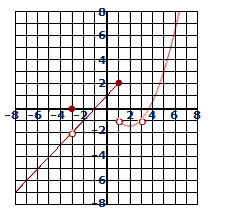# Use the graph of H shown to find the limit. If necessary, state that the limit does not exist....

## Question:

Use the graph of {eq}H {/eq} shown to find the limit. If necessary, state that the limit does not exist.

{eq}\lim\limits_{x \rightarrow -1} H(x) {/eq}## Finding a Limit from a Graph:

Given a graph of a function {eq}f {/eq}, if as the independent variable in the graph approaches a given number {eq}b {/eq}, the {eq}y- {/eq}coordinate of the graph approaches a value {eq}y=c {/eq}, we conclude that the limit of {eq}f {/eq} at {eq}b {/eq} equals {eq}c {/eq}. That is, {eq}\displaystyle \lim_{x \to b} f(x)=c {/eq}.

## Answer and Explanation:

It is clear that the graph crosses the {eq}x- {/eq}axis at {eq}x=\,-1 {/eq}. That is, as {eq}x {/eq} approaches {eq}-1 {/eq}, the function {eq}H(x) {/eq} approaches {eq}0 {/eq}. Hence {eq}\lim\limits_{x \rightarrow -1} H(x)=0 {/eq}